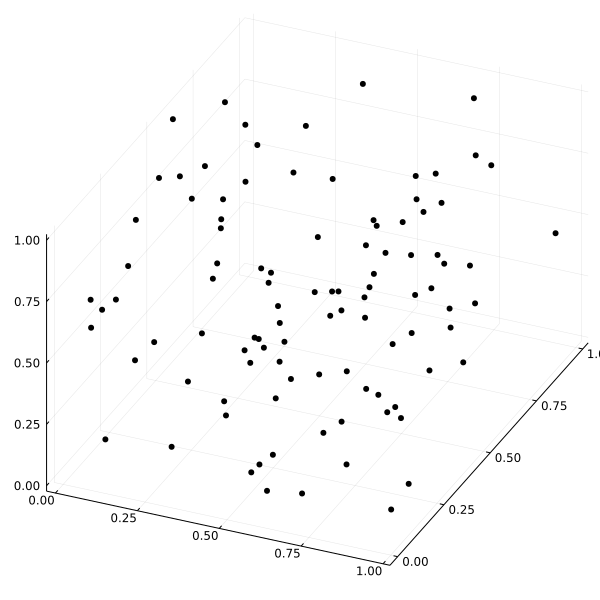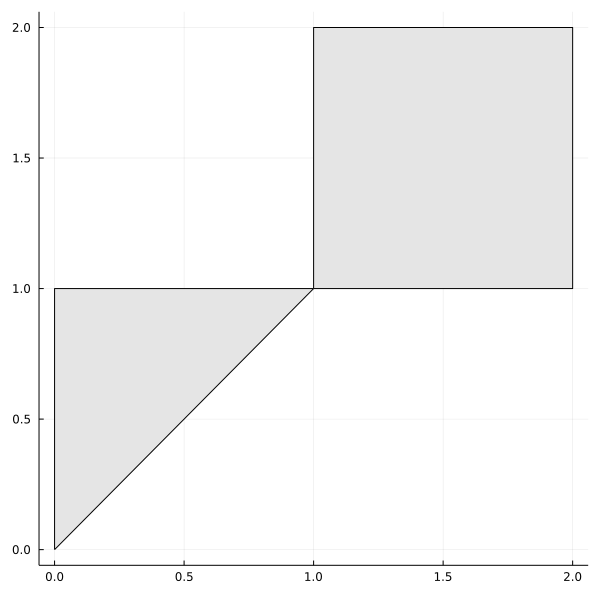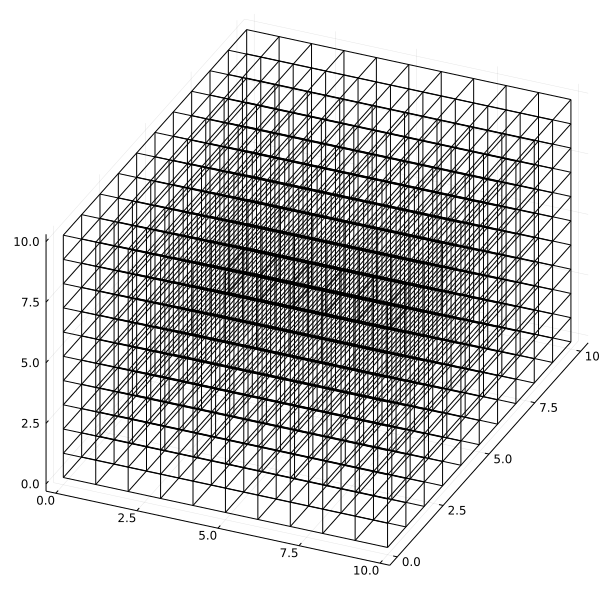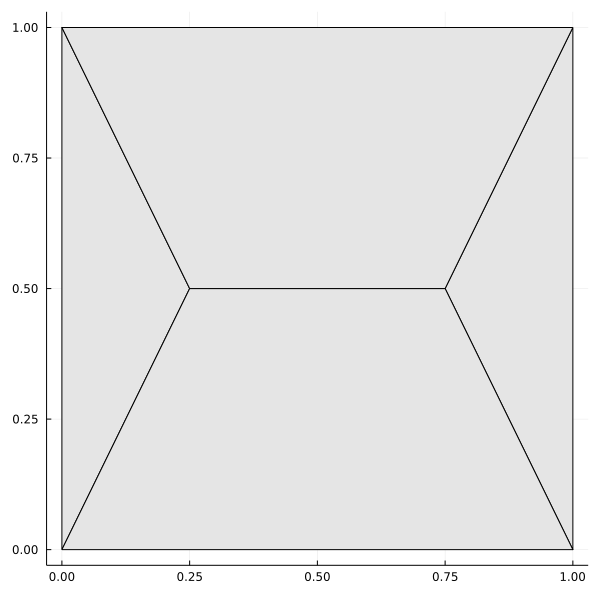Domains

A geospatial domain is a region in physical space where data can be observed. For example, a collection of rain gauge stations can be represented as a point set in the map. Similarly, a collection of states in a given country can be represented as a set of 2D shapes.

We provide flexible domain types for advanced geospatial workflows via the Meshes.jl project.

PointSet

Meshes.PointSetType
PointSet(points)

A set of points (a.k.a. point cloud) seen as a single domain.

Examples

All point sets below are the same and contain two points in R³:

julia> PointSet([Point(1,2,3), Point(4,5,6)])
julia> PointSet(Point(1,2,3), Point(4,5,6))
julia> PointSet([(1,2,3), (4,5,6)])
julia> PointSet((1,2,3), (4,5,6))
julia> PointSet([[1,2,3], [4,5,6]])
julia> PointSet([1,2,3], [4,5,6])
julia> PointSet([1 4; 2 5; 3 6])
plot(PointSet(rand(3,100)), camera=(30,60))GeometrySet

Meshes.GeometrySetType
GeometrySet(geometries)

A set of geometries seen as a single domain.

t = Triangle((0.0,0.0), (1.0,1.0), (0.0,1.0))
q = Quadrangle((1.0,1.0), (2.0,1.0), (2.0,2.0), (1.0,2.0))

gset = GeometrySet([t, q])
2 GeometrySet{2,Float64}
└─Triangle(Point(0.0, 0.0), Point(1.0, 1.0), Point(0.0, 1.0))
└─Quadrangle(Point(1.0, 1.0), Point(2.0, 1.0), Point(2.0, 2.0), Point(1.0, 2.0))
plot(gset, fillcolor=:gray90, linecolor=:black)CartesianGrid

Meshes.CartesianGridType
CartesianGrid(dims, origin, spacing)

A Cartesian grid with dimensions dims, lower left corner at origin and cell spacing spacing. The three arguments must have the same length.

CartesianGrid(start, finish, dims=dims)

Alternatively, construct a Cartesian grid from a start point (lower left) to a finish point (upper right).

CartesianGrid(start, finish, spacing)

Alternatively, construct a Cartesian grid from a start point to a finish point using a given spacing.

CartesianGrid(dims)
CartesianGrid(dim1, dim2, ...)

Finally, a Cartesian grid can be constructed by only passing the dimensions dims as a tuple, or by passing each dimension dim1, dim2, ... separately. In this case, the origin and spacing default to (0,0,...) and (1,1,...).

Examples

Create a 3D grid with 100x100x50 locations:

julia> CartesianGrid(100,100,50)

Create a 2D grid with 100x100 locations and origin at (10.,20.) units:

julia> CartesianGrid((100,100),(10.,20.),(1.,1.))

Create a 1D grid from -1 to 1 with 100 locations:

julia> CartesianGrid((-1.,),(1.,), dims=(100,))
plot(CartesianGrid(10,10,10), camera=(30,60))SimpleMesh

Meshes.SimpleMeshType
SimpleMesh(points, connec)

A simple mesh with points and connectivities connec. The i-th face of the mesh is lazily built based on the connectivity list connec[i].

SimpleMesh(points, topology)

Alternatively, construct a simple mesh with points and a topological data structure (e.g. HalfEdgeTopology).

points = Point2[(0,0), (1,0), (0,1), (1,1), (0.25,0.5), (0.75,0.5)]
connec = connect.([(1,5,3),(4,6,2),(1,2,6,5),(4,3,5,6)], Ngon)
mesh   = SimpleMesh(points, connec)
4 SimpleMesh{2,Float64}
6 vertices
└─Point(0.0, 0.0)
└─Point(1.0, 0.0)
└─Point(0.0, 1.0)
└─Point(1.0, 1.0)
└─Point(0.25, 0.5)
└─Point(0.75, 0.5)
4 elements
└─Triangle(1, 5, 3)
└─Triangle(4, 6, 2)